Published © GPL3+

# Windows IoT Core Oscilloscope

Interesting oscilloscope project using a Windows IoT Core-based Raspberry Pi 2 and a simple ADC.

IntermediateFull instructions provided4,438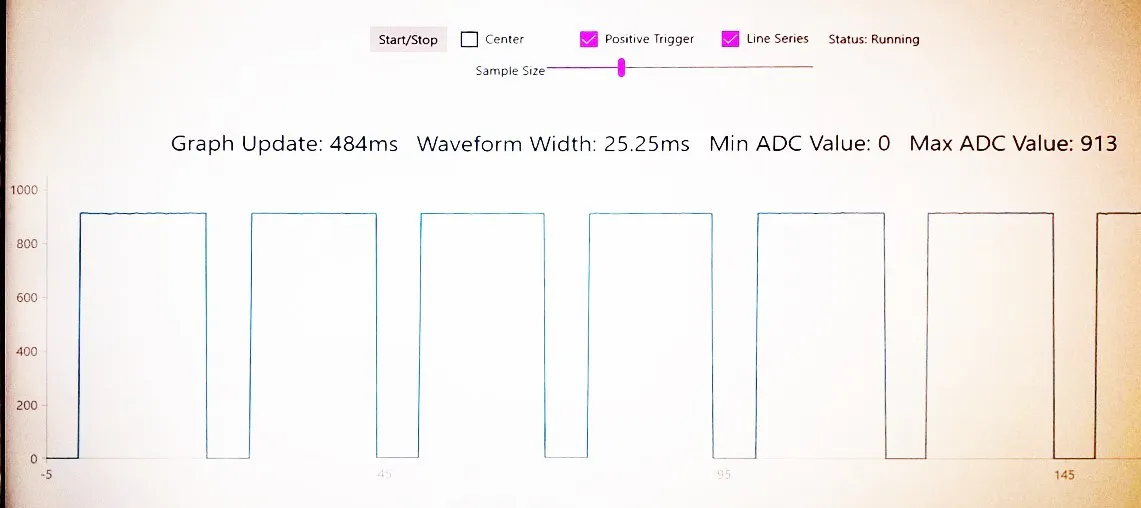## Things used in this project

### Hardware components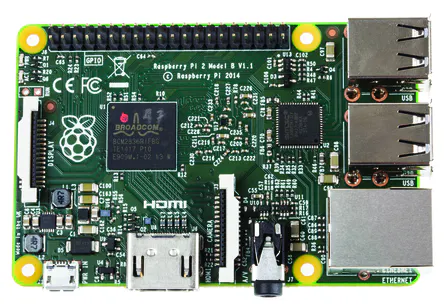Raspberry Pi 2 Model B
×1
 MCP3002
×1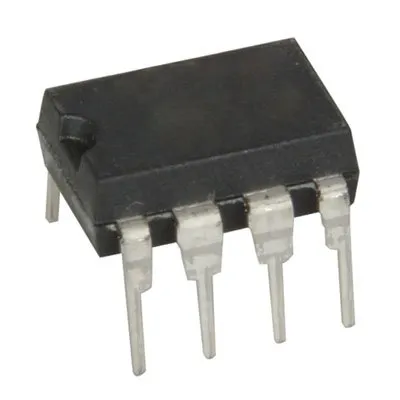555 or 556 Timer (generic)
×1

### Software apps and online servicesMicrosoft Visual Studio 2015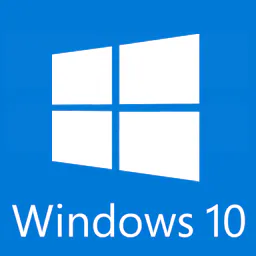Microsoft Windows 10 IoT Core

Read more

## Schematics

### Windows IoT Core Oscilloscope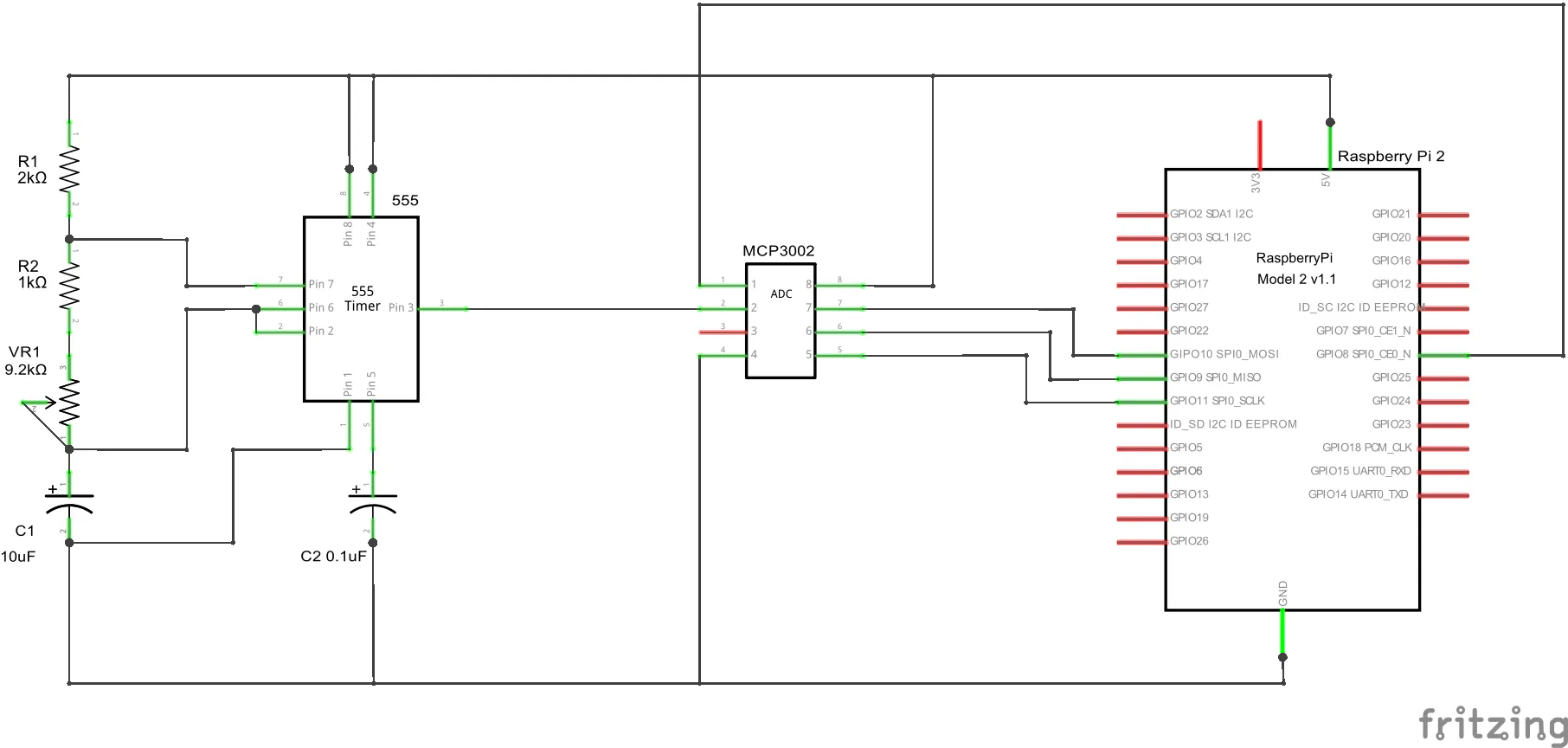## Code

### Initialization

C#
The initialization of the ADC is handled with the following calls. Note that we can select between the MCP3002 and MCP3208 with the AdcDevice enum.
```enum AdcDevice { NONE, MCP3002, MCP3208 };
private AdcDevice ADC_DEVICE = AdcDevice.MCP3002;
private const string SPI_CONTROLLER_NAME = "SPI0";  /* Friendly name for Raspberry Pi 2 SPI controller          */
private const Int32 SPI_CHIP_SELECT_LINE = 0;       /* Line 0 maps to physical pin number 24 on the Rpi2        */
private SpiDevice SpiADC;

private async void InitAll()
{
if (ADC_DEVICE == AdcDevice.NONE)
{
viewModel.Status = "Please change the ADC_DEVICE variable to either MCP3002 or MCP3208";
return;
}

try
{
await InitSPI();    /* Initialize the SPI bus for communicating with the ADC      */
}
catch (Exception ex)
{
viewModel.Status = ex.Message;
return;
}

running = true;
periodicTimer = new Timer(this.Running_Timer_Tick, null, 0, System.Threading.Timeout.Infinite);
viewModel.Status = "Status: Running";
}

private async Task InitSPI()
{
try
{
var settings = new SpiConnectionSettings(SPI_CHIP_SELECT_LINE);
settings.ClockFrequency = 500000;   /* 0.5MHz clock rate                                        */
settings.Mode = SpiMode.Mode0;      /* The ADC expects idle-low clock polarity so we use Mode0  */

string spiAqs = SpiDevice.GetDeviceSelector(SPI_CONTROLLER_NAME);
var deviceInfo = await DeviceInformation.FindAllAsync(spiAqs);
SpiADC = await SpiDevice.FromIdAsync(deviceInfo.Id, settings);
}

/* If initialization fails, display the exception and stop running */
catch (Exception ex)
{
throw new Exception("SPI Initialization Failed", ex);
}
}
```

### Signal Capture

C#
The capture portion of the code is listed below.
Note that we use the Stepwatch.Frequency value to calculate the Tick to millisecond factor. That way we can convert the tick value to an actual millisecond value. We use the first detected zero crossing as the 0 millisecond timestamp that the entire dataset is based upon. This keeps sucessive repeating waveforms lined up on the graph. The processed data is pushed into the view model so that the UI can be updated.
```private void Running_Timer_Tick(object state)
{
periodicTimer.Dispose();
TakeReadings();
if (running)
{
periodicTimer = new Timer(this.Running_Timer_Tick, null, interval, System.Threading.Timeout.Infinite);
}
}

private void TakeReadings()
{
SampleValue[] samples = new SampleValue[sampleSize];
ReadFast(samples);
int average = 0;
int min = int.MaxValue;
int max = int.MinValue;
OscopeHelper.ProcessStats(samples, ref average, ref min, ref max);
if (normalized)
{
OscopeHelper.NormalizeToAverage(samples, (min + max) / 2);
}
var crossings = OscopeHelper.ProcessZeroCrossings(samples, positiveTrigger, normalized ? 0 : 512, 2, 1);
double cross = crossings.Any() ? crossings.First().Value : 0;
double oneCycle = crossings.Count > 1 ? (crossings.ElementAt(1).Value - crossings.ElementAt(0).Value) : 0;

var task2 = this.Dispatcher.RunAsync(Windows.UI.Core.CoreDispatcherPriority.Normal, () =>
{
var freq = 1000.0 / Stopwatch.Frequency;
viewModel.Points = samples.Select(s => new ScatterDataPoint
{
XValue = (s.Tick - cross) * freq,
YValue = s.Value,
});
viewModel.Average = average;
viewModel.Min = min;
viewModel.Max = max;
viewModel.OneCycleMS = oneCycle * freq;
var now = DateTime.Now;
viewModel.RunMS = (now - lastRun).TotalMilliseconds;
lastRun = now;
});
}
```

### SampleValue

C#
The TakeReadings method handles the entire signal capture and processing process. The raw ADC values are stored in a SampleValue array.
```public class SampleValue
{
public long Tick { get; set; }
public int Value { get; set; }
}
```

### Sampling

C#
The ReadFast method is where we actually capture the ADC data. Note the ADC_DEVICE enum is used to select and process the data from the correct ADC chip. The for loop is where we actually read the values from the ADC. We're using a Stopwatch object to get the timestamp. The DateTime.Now or DateTime.UtcNow does not have the millisecond resolution we need. Those calls only get updated by the system every 16 milliseconds at best. The Stopwatch.GetTimestamp call is updated at each call and returns the raw system Ticks.
```private void ReadFast(SampleValue[] samples)
{
byte[] readBuffer = new byte; /* Buffer to hold read data*/
byte[] writeBuffer = new byte { 0x00, 0x00, 0x00 };
Func<byte[], int> convertToIntFunc = null;
/* Setup the appropriate ADC configuration byte */
switch (ADC_DEVICE)
{
case AdcDevice.MCP3002:
writeBuffer = MCP3002_CONFIG;
convertToIntFunc = convertToIntMCP3002;
break;
case AdcDevice.MCP3208:
writeBuffer = MCP3208_CONFIG;
convertToIntFunc = convertToIntMCP3208;
break;
}
Stopwatch.StartNew();
int sampleSize = samples.Length;
for (int sampleIndex = 0; sampleIndex < sampleSize; sampleIndex++)
{
SpiADC.TransferFullDuplex(writeBuffer, readBuffer); /* Read data from the ADC                           */
adcValue = convertToIntFunc(readBuffer);            /* Convert the returned bytes into an integer value */
samples[sampleIndex] = new SampleValue() { Tick = Stopwatch.GetTimestamp(), Value = adcValue };
}
}
```

### Convert

C#
We use the converToIntFunc call to correctly convert the readBuffer depending upon the selected ADC enum. Doing these calls in the capture loop or outside after the loop completed made no appreciatable difference in the capture speed so I kept them in for simplicity.
```public int convertToIntMCP3002(byte[] data)
{
int result = data & 0x03;
result <<= 8;
result += data;
return result;
}

public int convertToIntMCP3208(byte[] data)
{
int result = data & 0x0F;
result <<= 8;
result += data;
return result;
}
```

### ProcessStats

C#
There are a few helper functions in the TakeReadings method that handle various signal processing functions. The ProcessStats method calculates the min, max, and average of the entire sample set.
```public static void ProcessStats(SampleValue[] samples, ref int average, ref int min, ref int max)
{
average = 0;
int sampleSize = samples.Length;
for (int i = 0; i < sampleSize - 1; i++)
{
if (samples[i].Value < min)
{
min = samples[i].Value;
}
if (samples[i].Value > max)
{
max = samples[i].Value;
}
average += samples[i].Value;
}
average = average / sampleSize;
}
```

### NormalizeToAverage

C#
The NormalizeToAverage method adjusts the data in the SampleValue array to be centered upon the average value.
```public static void NormalizeToAverage(SampleValue[] samples, int average)
{
int sampleSize = samples.Length;
for (int i = 1; i < sampleSize; i++)
{
samples[i].Value = samples[i].Value - average;
}
}
```

### ProcessZeroCrossing

C#
The ProcessZeroCrossing function returns a dictionary of all of the detected zero crossings that the waveform goes through. It will process positive or negative crossing depending upon the positiveTrigger parameter. The offset parameter is the level that is used to detect the crossing. Normally this is the average of the signal but can be anything depending upon how you want to view the signal. The crossingCount parameter can be used to limit the number of crossings detected. Leaving it null will process the entire dataset. The averageCount parameter is used to perform a simple average filter on the dataset. The value passed is the number of points to average for each index.
```public static Dictionary<int, long> ProcessZeroCrossings(
SampleValue[] samples,
bool positiveTrigger = false,
int offset = 0,
int? crossingsCount = null,
int averageCount = 4)
{
AverageValue averageValue = new AverageValue(averageCount);
var crossings = new Dictionary<int, long>();
bool crossSet = false;
int sampleSize = samples.Length;
for (int i = 0; i < sampleSize; i++)
{
averageValue.Value = samples[i].Value;
if (i > averageValue.SampleSize)
{
var fvalue = averageValue.Value;
var averageIndex = i - averageValue.SampleSize / 2;
samples[averageIndex].Value = (int)fvalue;
if (crossingsCount.HasValue && crossings.Count < crossingsCount.Value)
{
if (!crossSet && ((!positiveTrigger && (fvalue > offset)) || (positiveTrigger && (fvalue < offset))))
{
crossSet = true;
}
if (crossSet && ((!positiveTrigger && (fvalue < offset)) || (positiveTrigger && (fvalue > offset))))
{
crossings.Add(averageIndex, samples[i].Tick);
crossSet = false;
}
}
}
}
return crossings;
}
```

### AverageValue

C#
The averaging is handled by the AverageValue object. This uses a Queue object to handle a running average value of the signal.
```public class AverageValue
{
Queue queue;
double _Sum;
int _SampleSize;

public AverageValue(int samplesize = 5)
{
SampleSize = samplesize;
}

public int SampleSize
{
get { return _SampleSize; }
set
{
if (value > 0)
{
_SampleSize = value;
queue = new Queue(_SampleSize);
}
}
}

public double Value
{
get { return _Sum / queue.Count; }
set
{
if (queue.Count == _SampleSize)
{
_Sum -= (double)queue.Dequeue();
}
queue.Enqueue(value);
_Sum += value;
}
}
}
```

### XAML

XML
The last bit of code that we'll cover is the XAML. We're using a Telerik RadCartesianChart to do the graph.
```<Page
x:Class="IoTOscilloscope.MainPage"
xmlns="http://schemas.microsoft.com/winfx/2006/xaml/presentation"
xmlns:x="http://schemas.microsoft.com/winfx/2006/xaml"
xmlns:local="using:IoTOscilloscope"
xmlns:utilities="using:Falafel.Utilities"
xmlns:telerikChart="using:Telerik.UI.Xaml.Controls.Chart"
xmlns:d="http://schemas.microsoft.com/expression/blend/2008"
xmlns:mc="http://schemas.openxmlformats.org/markup-compatibility/2006"
mc:Ignorable="d">
<Page.DataContext>
<local:MainViewModel />
</Page.DataContext>
<Page.Resources>
<utilities:VisibilityConverter x:Key="visibility" />
<utilities:VisibilityConverter x:Key="invisibility" Inverse="True" />
<utilities:StringFormatValueConverter x:Key="stringFormatValueConverter" />
</Page.Resources>

<Grid Background="{ThemeResource ApplicationPageBackgroundThemeBrush}">
<StackPanel HorizontalAlignment="Center" VerticalAlignment="Center">
<StackPanel Orientation="Horizontal" HorizontalAlignment="Center">
<Button Click="Button_Click" Content="Start/Stop"/>
<CheckBox Content="Center" IsChecked="{Binding Normalize, Mode=TwoWay}" Margin="15,0,0,0"/>
<CheckBox Content="Positive Trigger" IsChecked="{Binding PositiveTrigger, Mode=TwoWay}" Margin="15,0,15,0"/>
<CheckBox Content="Line Series" IsChecked="{Binding LineSeries, Mode=TwoWay}" Margin="15,0,0,0"/>
<TextBlock x:Name="StatusText" Text="{Binding Status}" VerticalAlignment="Center" />
</StackPanel>
<StackPanel Orientation="Horizontal" HorizontalAlignment="Center">
<TextBlock Text="Sample Size" VerticalAlignment="Center"/>
<Slider Width="300" Minimum="200" Maximum="2000" StepFrequency="100" Value="{Binding SampleSize, Mode=TwoWay}" />
</StackPanel>
<StackPanel Orientation="Horizontal" HorizontalAlignment="Center">
<TextBlock x:Name="runMSText" Text="{Binding RunMS, Converter={StaticResource stringFormatValueConverter}, ConverterParameter='Graph Update: \{0:0\}ms'}" Margin="10,50,10,10" TextAlignment="Center" FontSize="26.667" />
<TextBlock x:Name="oneCycleText" Text="{Binding OneCycleMS, Converter={StaticResource stringFormatValueConverter}, ConverterParameter='Waveform Width: \{0:0.00\}ms'}" Margin="10,50,10,10" TextAlignment="Center" FontSize="26.667" />
<TextBlock x:Name="minText" Text="{Binding Min, Converter={StaticResource stringFormatValueConverter}, ConverterParameter='Min ADC Value: \{0:0\}'}" Margin="10,50,10,10" TextAlignment="Center" FontSize="26.667" />
<TextBlock x:Name="maxText" Text="{Binding Max, Converter={StaticResource stringFormatValueConverter}, ConverterParameter='Max ADC Value: \{0:0\}'}" Margin="10,50,10,10" TextAlignment="Center" FontSize="26.667" />
</StackPanel>
<telerikChart:RadCartesianChart HorizontalAlignment="Stretch" VerticalAlignment="Top" x:Name="dataChart" Height="400">
<telerikChart:RadCartesianChart.VerticalAxis>
<telerikChart:LinearAxis Minimum="{Binding GraphMin}" Maximum="{Binding GraphMax}"/>
</telerikChart:RadCartesianChart.VerticalAxis>
<telerikChart:RadCartesianChart.HorizontalAxis>
<telerikChart:LinearAxis Minimum="-5" Maximum="{Binding GraphXMax}"/>
</telerikChart:RadCartesianChart.HorizontalAxis>
<telerikChart:ScatterPointSeries ItemsSource="{Binding Points}" Visibility="{Binding LineSeries, Converter={StaticResource invisibility}}">
<telerikChart:ScatterPointSeries.XValueBinding>
<telerikChart:PropertyNameDataPointBinding PropertyName="XValue"/>
</telerikChart:ScatterPointSeries.XValueBinding>
<telerikChart:ScatterPointSeries.YValueBinding>
<telerikChart:PropertyNameDataPointBinding PropertyName="YValue"/>
</telerikChart:ScatterPointSeries.YValueBinding>
</telerikChart:ScatterPointSeries>
<telerikChart:ScatterLineSeries ItemsSource="{Binding Points}" Visibility="{Binding LineSeries, Converter={StaticResource visibility}}">
<telerikChart:ScatterLineSeries.XValueBinding>
<telerikChart:PropertyNameDataPointBinding PropertyName="XValue"/>
</telerikChart:ScatterLineSeries.XValueBinding>
<telerikChart:ScatterLineSeries.YValueBinding>
<telerikChart:PropertyNameDataPointBinding PropertyName="YValue"/>
</telerikChart:ScatterLineSeries.YValueBinding>
</telerikChart:ScatterLineSeries>
</telerikChart:RadCartesianChart>
</StackPanel>
</Grid>
</Page>
```

### Github

https://github.com/FalafelSoftwareInc/IoTCoreOscilloscope

## Credits

### Bary Nusz

8 projects • 32 followers
Nusz Labs “Tinkerer-in-Chief”.